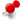# Render latex in tableClassicListThreaded3 messagesOpen this post in threaded view
|

## Render latex in table

 # Description I want to use asciidoctor table to record some formulas in probability theory, some formulas involves latex.  |=== | distribution | parameter | probability distribution | E(x) | D(x) | binominal distribution | n \geqslant 1 0 < p < 1 | P{X=k} = \binom{n}{k} p^k (1-p)^{n-k} | np | np(1-p) | poisson distribution | \lambda > 0 | P{X=k} = \frac{\lambda^k e^{-\lambda}}{k!}, k=0,1 ... | \lambda | \lambda | uniform distribution | a < b | | \frac{a+b}{2} | \frac{(b-a)^2}{12} | exponential distribution | \lambda > 0 | | \frac{1}{\lambda} | \frac{1}{\lambda^2} | normal distribution | \mu, \sigma > 0 | | \mu | \sigma^2 |===  # First try failed I go through the Asciidoctor User Manual, and decide to use stem block  = Render formula in table :stem: latexmath [stem] ++++ put that table here ++++  But it doesn't render table. # Second try success Then I try to use inline latexmath macro syntax like latexmath:[C = \alpha + \beta Y^{\gamma} + \epsilon]  = Render formula in table :stem: latexmath [stem] ++++ |=== | distribution | parameter | probability distribution | E(x) | D(x) | binominal distribution | n \geqslant 1 0 < p < 1 | latexmath:[P{X=k} = \binom{n}{k} p^k (1-p)^{n-k}] | np | np(1-p) |=== ++++  It works, but I need to add inline latexmath macro syntax for every formula. # Better way? I want to know if there is some easier ways to do that?